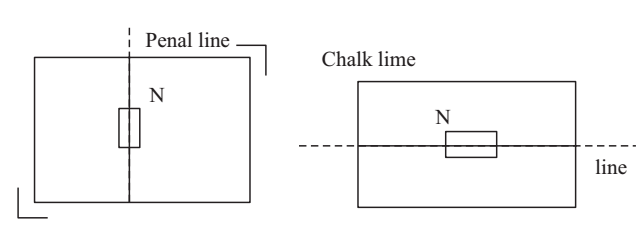# Lines of Force of Bar Magnet Experiment

Experiment: To draw the lines of force due to a bar magnet keep (i) N-pole pointing to magnetic north of the earth (ii) S-pole pointing to magnetic north of the earth. Locate neutral points.

The common bar magnet is a magnetized piece of iron. It has maximum attracting power near the ends. These are called the poles. To find which end is N and which is S, it is suspended freely with the help of a thread tied in the middle.

After some time it will come to rest in N-S direction. The end which points toward geographic North is called N-pole and the other is S-pole. The line joining N and S passing through the middle of magnet is usual its magnetic axis.

At a point in space around the bar magnet, where there are two equal opposite magnetic fields cancelling each other, there is a neutral point. Here there will be no magnetic field. In this experiment, one of the two fields is produced by the bar magnet and the other is earth’s horizontal magnetic field. These two combine together to give the neutral point.

The lines of force are the paths on which a hypothetical N-pole set free will move in the given magnetic field. These are supposed to come out of N-pole and enter the S-pole and form closed lines. These are curves around the bar magnet.

The line of symmetry AB, which is a straight line of force passing through the poles is the magnetic axis of the magnet. Earth’s magnetic field being uniform in the small region of your laboratory, gives parallel lines of force.

Material Required

Two bar magnets, compass needle, white paper, drawing board, drawing pins, pencil, chalk.

### How To Set Up

1. Find the N-pole-of the bar magnet and mark the end with ink.

2. Fix a white paper on the drawing board.

3. Draw a line in pencil through the middle of paper along the short edge for performing the first part of experiment i.e. N-pole of magnet toward north. For the second part of the experiment line will have to be drawn parallel to long edge.4. Place the magnet in the middle of line also shown above.

5. Take a small compass box and place it on a wooden table. Place a metre rod beside it so that it is parallel to the needle. Remove the compass and draw a line with a chalk. This line gives the magnetic meridian at the place.

6. Place the drawing board such that the line on paper in pencil is parallel to the line drawn in chalk on the table.

### How To Perform Experiment

A. N-Pole facing North

1. After placing the magnet, mark the boundary of board in chalk, so that its position is not displaced during the experiment. If at all it accidentally gets displaced, it can be put back in its original position.

2. Take a small compass box. Place it near the N-pole of the bar magnet with it pointer pointing towards the pencil dot marked near the N-pole. Mark the dot on the other side of needle. Move the compass box to the second marked dot, again mark a dot near the far end of needle. Repeat this process till you reach the S-pole. You will get a chain of dots which can be joined by a smooth curved line.

3. Join these dots with free hand. This gives the line of force. Mark arrow head on it pointing away for N-pole as shown.

4. Draw such lines for different starting points and you will get large number of lines of force around the magnet.

These lines will not cut each other. You will get two regions on the equatorial line, where there will be no line of force. These are the neutral points. There are two neutral points, one on each side of the magnet.

If you place the compass here in the circle with its centre at the neutral point, the needle wire not point in any fixed direction. It can come to rest in any orientation. That shows that no force is acting on it. If magnet is properly placed, each of these points will be equidistant from the two poles and lie exactly on equatorial line.

B. N-Pole facing South

1. For drawing the magnetic field in this case and locating neutral points place the drawing board with pencil line on paper parallel to long edge of board, along the N-S line of earth.

2. Now follow the same procedure as in the last experiment.

Here, the two neutral points are located on the axial line of the magnet. Because it is at these points where the earth’s horizontal field and the magnetic field of magnet balance each other.

### Sources of Error

1. The magnet may not be placed symmetrically not the line in pencil on paper.
2. The N-S line drawn on the table may not be correct. Due to both these errors the field drawn is not symmetrical about the line in pencil drawn on the paper.